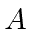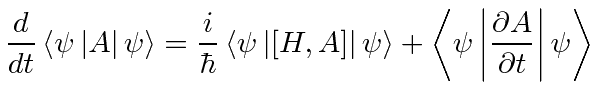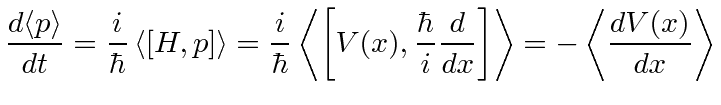## Time Derivative of Expectation Values *

We wish to compute the time derivative of the expectation value of an operatorin the state. Thinking about the integral, this has three terms.This is an important general result for the time derivative of expectation values.which becomes simple if the operator itself does not explicitly depend on time.Expectation values of operators that commute with the Hamiltonian are constants of the motion.

We can apply this to verify that the expectation value ofbehaves as we would expect for a classical particle.This is a good result. This is called the Ehrenfest Theorem.

For momentum,which Mr. Newton told us a long time ago.

Jim Branson 2013-04-22